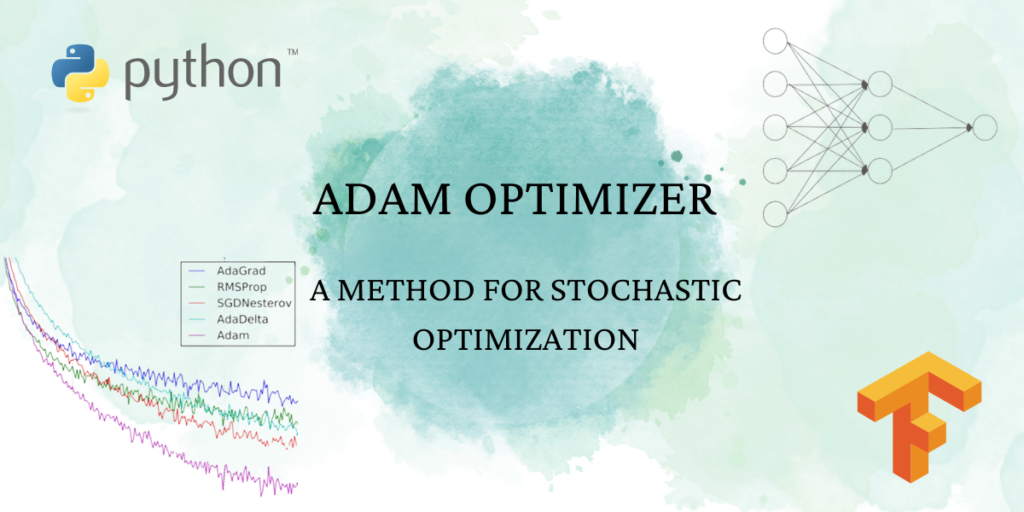# Adam optimizer: A Quick IntroductionOptimization is one of the critical processes in deep learning that helps in tuning the parameters of a model to minimize the loss function. Adam optimizer is one of the widely used optimization algorithms in deep learning that combines the benefits of Adagrad and RMSprop optimizers.

In this article, we will discuss the Adam optimizer, its features, and an easy-to-understand example of its implementation in Python using the Keras library.

## What is Adam Optimizer and how it works?

Adam stands for Adaptive Moment Estimation. It is an optimization algorithm that was introduced by Kingma and Ba in their 2014 paper. The algorithm computes the adaptive learning rates for each parameter and stores the first and second moments of the gradients.

Adam optimizer is an extension of the stochastic gradient descent (SGD) algorithm that updates the learning rate adaptively. The Adam optimizer updates the parameters of the model using the first and second moments of the gradients. The first moment is the mean of the gradients, and the second moment is the uncentered variance of the gradients.

The algorithm computes the adaptive learning rates for each parameter and uses the first and second moments of the gradients to adapt the learning rate. This helps in providing a different learning rate for each parameter and hence more precise parameter updates.

The working of Adam optimizer can be summarized in the following steps:

1. Initialize the learning rate and the model weights.
2. Compute the gradients of the model with respect to the loss function using backpropagation.
3. Compute the moving average of the gradient and the squared gradient.
4. Compute the bias-corrected moving averages.
5. Update the model weights using the bias-corrected moving averages.

The Adam optimizer updates the learning rate adaptively, depending on the gradient’s moving average and the squared gradient’s moving average. The moving average is computed for each parameter, and the learning rate is updated accordingly. This helps in providing a different learning rate for each parameter, which is useful in case some parameters are more sensitive than others.

Let’s understand this with an easy to understand example:

Minimize the value of function x^3 – 2*x^2 + 2. The manual computation looks something like this:

## Adam Optimizer Implementation in Python

Now let’s see how to use Adam optimizer for computing the same:

```import tensorflow as tf
import numpy as np
import matplotlib.pyplot as plt

# Define the function
def f(x):
return x**3 - 2*x**2 + 2

# Define the starting point for optimization
x = tf.Variable(0.001)

# Define a list to store the history of x values
x_values = []

# Define the number of optimization steps
num_steps = 100

# Perform the optimization
for i in range(num_steps):
# Calculate the value of the function and record the gradient
y = f(x)
# Use the Adam optimizer to update the value of x
# Record the current value of x
x_values.append(x.numpy())

# Print the optimized value of x and the value of the function at that point
print("Optimized value of x:", x.numpy())
print("Value of the function at the optimized point:", f(x.numpy()))
# Plot the function and the optimization path
x_plot = np.linspace(-2, 2, 500)
y_plot = f(x_plot)
plt.plot(x_plot, y_plot, label='Function')
plt.plot(x_values, [f(x) for x in x_values], label='Optimization path', marker='o')
plt.xlabel('x')
plt.ylabel('y')
plt.title('Function and Optimization Path')
plt.legend()
plt.show()
```

The output looks like this:

Now that we’ve understood the working of Adam with an example, let’s also know about how it is different from other optimizers.

Let’s see how is this different from other optimizers:

• Adam optimizer computes the adaptive learning rates for each parameter, which aids in quicker convergence and better generalization. This means that the learning rate adjusts during training, based on historical gradient information. It differs from stochastic gradient descent, which uses a fixed learning rate.
• The first and second moments of gradients are stored in Adam optimizer, reducing gradient noise and enhancing optimization algorithm stability. This is distinct from stochastic gradient descent, which stores no historical gradient information.
• Adam optimizer is sturdy against noisy gradients, handling non-stationary objectives and sidestepping local minima and saddle points. In contrast, stochastic gradient descent may get stuck in local minima.